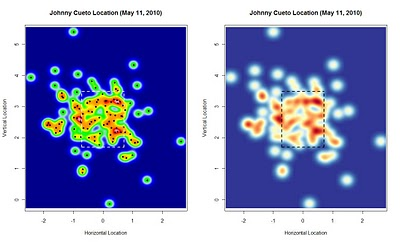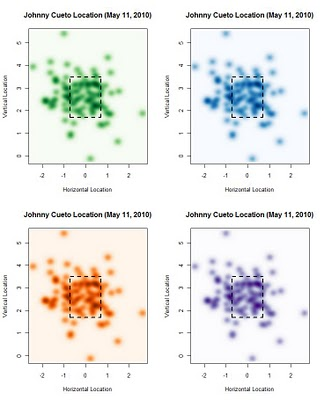Want to share your content on R-bloggers? click here if you have a blog, or here if you don't.

I tried using my own little palette with more traditional looking heatmap colors (red and pink are the densest, blue and green are less so, yellow in the middle, etc.). I also included the actual points, but would recommend it for more than a single game or two worth of pitches. I provide the code to create this same plot (just remember that it depends on how your data is structured in terms of what the points are actually plotting). One more thing to keep in mind: if you have more points, each single pitch will have less of an impact on the color in the surrounding area. For example, you won’t have those big white blotches I have below for single pitches if you’re plotting an entire season…so if you don’t like those, then no worries.

Just curious which one others think looks better. I really like the colors on the one on the right, but the one on the left is also interesting. The right plot seems to have some blurriness to it that goes along with the color palette, while the other one is less so. My opinion is that the colors don’t blend as well in the plot on the left. Part of the fun with this is finding the right balance of colors.library(graphics)
par(mfrow=c(1,2))
smoothScatter(data\$pz~data\$px, nbin=1000, colramp = colorRampPalette(c(“darkblue”, “blue”, “lightblue”, “green”, “yellow”, “orange”, “darkorange”, “red”, “pink”)), nrpoints=Inf, pch=19, cex=.7, transformation = function(x) x^.75, col=”black”, main=”Johnny Cueto Location (May 11, 2010)”, xlab=”Horizontal Location”, ylab=”Vertical Location”)
lines(c(0.708335, 0.708335), c(mean(data\$sz_bot), mean(data\$sz_top)), col=”white”, lty=”dashed”, lwd=2)
lines(c(-0.708335, -0.708335), c(mean(data\$sz_bot), mean(data\$sz_top)), col=”white”, lty=”dashed”, lwd=2)
lines(c(-0.708335, 0.708335), c(mean(data\$sz_bot), mean(data\$sz_bot)), col=”white”, lty=”dashed”, lwd=2)
lines(c(-0.708335, 0.708335), c(mean(data\$sz_top), mean(data\$sz_top)), col=”white”, lty=”dashed”, lwd=2)

library(RColorBrewer)
buylrd <- c("#313695", "#4575B4", "#74ADD1", "#ABD9E9", "#E0F3F8", "#FFFFBF", "#FEE090", "#FDAE61", "#F46D43", "#D73027", "#A50026") smoothScatter(data\$pz~data\$px, nbin=1000, colramp = colorRampPalette(c(buylrd)), nrpoints=Inf, pch="", cex=.7, transformation = function(x) x^.6, col="black", main="Johnny Cueto Location (May 11, 2010)", xlab="Horizontal Location", ylab="Vertical Location") lines(c(0.708335, 0.708335), c(mean(data\$sz_bot), mean(data\$sz_top)), col="white", lty="dashed", lwd=2) lines(c(-0.708335, -0.708335), c(mean(data\$sz_bot), mean(data\$sz_top)), col="white", lty="dashed", lwd=2) lines(c(-0.708335, 0.708335), c(mean(data\$sz_bot), mean(data\$sz_bot)), col="white", lty="dashed", lwd=2) lines(c(-0.708335, 0.708335), c(mean(data\$sz_top), mean(data\$sz_top)), col="white", lty="dashed", lwd=2) And, for good measure, the same plots using a single color shaded differently based on density (the default for the smoothScatter function). Just for fun. I don't like these as much, and they look more like blurry scatter plots than anything else.